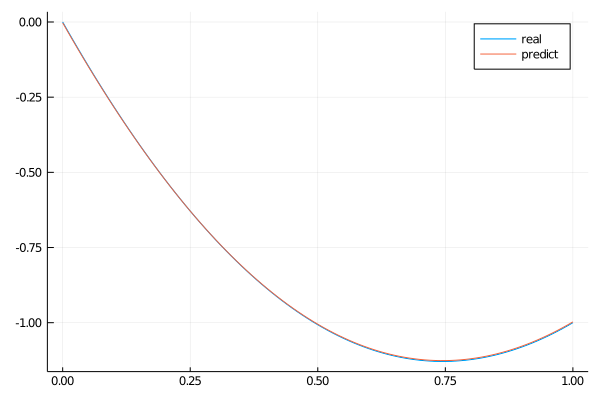# ODE with a 3rd-Order Derivative

Let's consider the ODE with a 3rd-order derivative:

\begin{align*} ∂^3_x u(x) &= \cos(\pi x) \, ,\\ u(0) &= 0 \, ,\\ u(1) &= \cos(\pi) \, ,\\ ∂_x u(0) &= 1 \, ,\\ x &\in [0, 1] \, , \end{align*}

We will use physics-informed neural networks.

using NeuralPDE, Lux, ModelingToolkit
using Optimization, OptimizationOptimJL, OptimizationOptimisers
import ModelingToolkit: Interval, infimum, supremum

@parameters x
@variables u(..)

Dxxx = Differential(x)^3
Dx = Differential(x)
# ODE
eq = Dxxx(u(x)) ~ cos(pi*x)

# Initial and boundary conditions
bcs = [u(0.) ~ 0.0,
u(1.) ~ cos(pi),
Dx(u(1.)) ~ 1.0]

# Space and time domains
domains = [x ∈ Interval(0.0,1.0)]

# Neural network
chain = Lux.Chain(Dense(1,8,Lux.σ),Dense(8,1))

discretization = PhysicsInformedNN(chain, QuasiRandomTraining(20))
@named pde_system = PDESystem(eq,bcs,domains,[x],[u(x)])
prob = discretize(pde_system,discretization)

callback = function (p,l)
println("Current loss is: \$l")
return false
end

res = Optimization.solve(prob, Adam(0.01); callback = callback, maxiters=2000)
phi = discretization.phi

We can plot the predicted solution of the ODE and its analytical solution.

using Plots

analytic_sol_func(x) = (π*x*(-x+(π^2)*(2*x-3)+1)-sin(π*x))/(π^3)

dx = 0.05
xs = [infimum(d.domain):dx/10:supremum(d.domain) for d in domains]
u_real  = [analytic_sol_func(x) for x in xs]
u_predict  = [first(phi(x,res.u)) for x in xs]

x_plot = collect(xs)
plot(x_plot ,u_real,title = "real")
plot!(x_plot ,u_predict,title = "predict")